# DAV Class 8 Maths Chapter 14 Brain Teasers Solutions

The DAV Maths Book Class 8 Solutions Pdf and DAV Class 8 Maths Chapter 14 Brain Teasers Solutions of Mensuration offer comprehensive answers to textbook questions.

## DAV Class 8 Maths Ch 14 Brain Teasers Solutions

Question 1A.
Tick (✓) the correct option.
(i) The volume of a cube of side 0.01 m in cm3 is
(a) 0.001
(b) 1
(c) 0.0001
(d) 0.000001
Solution:
Side of the cube, l = 0.01 m = 1 cm
The volume of the cube = l3
= (1)3 cm3
= 1 cm3
Thus, option (b) is the correct answer.

(ii) If the height of a cylinder is halved, its volume will be
(a) $$\frac{1}{2}$$ times
(b) $$\frac{1}{3}$$ times
(c) 2 times
(d) 3 times
Solution:
Let the radius of the cylinder be r, height be h and volume be V1.
Then, V1 = πr2h ……(i)
If the height of the cylinder becomes its half i.e., $$\frac{h}{2}$$
then its volume, say V2 = πr2 $$\frac{h}{2}$$ = $$\frac{\pi r^2 h}{2}$$ ……(ii)
From (i) and (ii), it is clear that volume becomes half when the height of the cylinder is halved.
Thus, option (a) is the correct answer.

(iii) The area of a trapezium having two parallel sides as 10 cm and 12 cm and height as 4 cm is
(a) 42 cm2
(b) 44 cm2
(c) 46 cm2
(d) 48 cm2
Solution:
Area of Trapezium = $$\frac{1}{2}$$ × height × sum of parallel sides
= $$\frac{1}{2}$$ × 4 × (10 + 12)
= $$\frac{1}{2}$$ × 4 × 22
= 44 cm2
Thus, option (b) is the correct answer.

(iv) The number of faces a tetrahedron has
(a) 14
(b) 12
(c) 6
(d) 4
Solution:
A tetrahedron has 4 faces. Thus, option (d) is the correct answer.

(v) If the length of the side of a cube is doubled, then the ratio of volumes of the new cube and the original cube is
(a) 1 : 2
(b) 2 : 1
(c) 4 : 1
(d) 8 : 1
Solution:
Let the side of a cube be l and its volume be V1.
Then, V1 = l3
Now, the side of a new cube be 2l and its volume be V2.
Then, V2 = (2l)3 = 8l3
∴ $$\frac{\mathrm{V}_2}{\mathrm{~V}_1}=\frac{8 l^3}{l^3}=\frac{8}{1}$$
∴ V2 : V1 = 8 : 1
Thus, option (d) is the correct answer.Question 1B.
(i) Find the number of edges of a polyhedron having 20 faces and 12 vertices.
Solution:
Here, F = 20, V = 12 and E = ?
Using Euler’s formula, we have
F + V – E = 2
⇒ 20 + 12 – E = 2
⇒ 32 – 2 = E
⇒ E = 30

(ii) Find the surface area of a cube whose volume is 729 m3.
Solution:
Let the side of the cube be l.
Then, the volume of the cube = l3
⇒ 729 = l3
⇒ 9 × 9 × 9 = l3
⇒ l3 = 93
⇒ l = 9 m
Surface area of the cube = 6l2
= 6 × 92
= 6 × 81
= 486 m2

(iii) The volume of a cylinder is 2,376 cm3. If the diameter of its base is 12 cm, then find its height.
Solution:
The base diameter of the cylinder = 12 cm
∴ The base radius of the cylinder = 6 cm
Let height of the cylinder be h cm.
Then, the volume of the cylinder = πr2h
2376 = $$\frac{22}{7}$$ × 6 × 6 × h (∵ π = $$\frac{22}{7}$$)
⇒ h = $$\frac{2376 \times 7}{22 \times 6 \times 6}$$
⇒ h = 21 cm

(iv) Draw the solid represented by the views given below:Solution: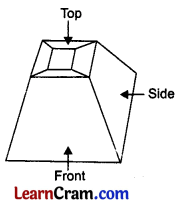(v) Find the total surface area of a cuboid of length 10 cm, breadth 8 cm, and height 6 cm.
Solution:
Given: l = 10 cm, b = 8 cm and h = 6 cm
Total surface area of a cuboid = 2(lb + bh + hl)
= 2{(10 × 8) + (8 × 6) + (6 × 10)}
= 2(80 + 48 + 60)
= 2 × 188
= 376 cm2Question 2.
Find the area of the given quadrilateral.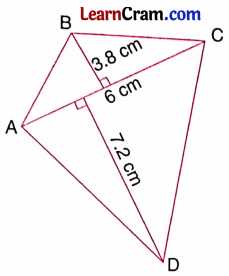Solution:
Area of the given quadrilateral = $$\frac{1}{2}$$ × AC × (BE + FD)
= $$\frac{1}{2}$$ × 6 × (3.8 + 7.2)
= $$\frac{1}{2}$$ × 6 × 11
= 33 cm2

Question 3.
A field is in the shape of a trapezium. One of its parallel sides is twice the other parallel side and distance between them is 100 m. If the area of the field is 10,500 m2, find the length of the parallel sides.
Solution:
Let one of the parallel sides be x m
∴ other parallel side = 2x m
Area of the trapezium = $$\frac{1}{2}$$ × (sum of parallel sides) × height
⇒ 10500 = $$\frac{1}{2}$$ × (x + 2x) × 100
⇒ 10500 = 3x × 50
⇒ x = $$\frac{10500}{3 \times 50}$$
⇒ x = 70 m
Hence, the two parallel sides are 70 m and 70 × 2 = 140 m

Question 4.
Two parallel sides DC and AB of a trapezium are 12 cm and 36 cm respectively. If nonparallel sides are each equal to 15 cm, find the area of the trapezium.
Solution: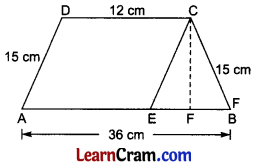Draw CE || DA.
∴ DA = CE = 15 cm
and EB = 36 – 12 = 24 cm
∴ EF = $$\frac{24}{2}$$ = 12 cm
∴ In right triangle CFE,
CE2 = EF2 + CF2 [From Pythagoras Theorem]
⇒ (15)2 = (12)2 + CF2
⇒ 225 = 144 + CF2
⇒ CF2 = 225 – 144
⇒ CF2 = 81
⇒ CF = 9
Area of trapezium ABCD = $$\frac{1}{2}$$ × (AB + DC) × CF
= $$\frac{1}{2}$$ × (36 + 12) × 9
= $$\frac{1}{2}$$ × 48 × 9
= 216 cm2Question 5.
The length, breadth, and height of a cuboidal box are 2 m 10 cm, 1 m, and 80 cm respectively. Find the area of canvas required to cover this box.
Solution:
Here, l = 2 m 10 cm = 2.1 m
b = 1 m
h = 80 cm = 0.8 m
∴ Surface area of the cuboidal box = 2[lb + bh + lh]
= 2[2.1 × 1 + 1 × 0.8 + 2.1 × 0.8]
= 2[2.1 + 0.8 + 1.68]
= 2[4.58]
= 9.16 m2

Question 6.
A metallic pipe is 0.7 cm thick. Inner radius of the pipe is 3.5 cm and length is 5 dm. Find its total surface area.
[Hint: Total surface area = Inner surface area + Outer surface area + Area of two rims]
Solution:
Here, inner radius r1 = 3.5 cm
thickness = 0.7 cm
∴ outer radius r2 = 3.5 + 0.7 = 4.2 cm
height h = 5 dm = 50 cm
∴ Total surface area = Inner surface area + Outer surface area + Area of two rims
= 2πr1h + 2πr2h + $$\pi\left(r_2^2+r_1^2\right)$$
= 2πh(r1 + r2) + $$\pi\left(r_2^2+r_1^2\right)$$
= 2 × $$\frac{22}{7}$$ × 50(3.5 + 4.2) + $$\frac{22}{7}$$ × (3.52 + 4.22)
= 2 × $$\frac{22}{7}$$ × 50 × 7.7 + $$\frac{22}{7}$$ × (12.25 + 17.64)
= 2 × $$\frac{22}{7}$$ × 50 × 7.7 + $$\frac{22}{7}$$ × 29.89
= $$\frac{22}{7}$$ × (2 × 50 × 7.7 + 29.89)
= $$\frac{22}{7}$$ × (770 + 29.89)
= $$\frac{22}{7}$$ × 799.89
= 2453.88 cm2

Question 7.
A cylinder with a curved surface area of 1250 m2 is formed from a rectangular metallic sheet. Find the dimensions of the rectangular sheet if its length is double its breadth.
Solution:
Let the breadth be x m.
∴ Length = 2x m
Area of the rectangular sheet = Curved surface of the sheet
⇒ 2x × x = 1250
⇒ 2x2 = 1250
⇒ x2 = $$\frac{1250}{2}$$ = 625
⇒ x = 25
Hence, length = 2 × 25 = 50 m and breadth = 25 m.

Question 8.
The diameter and length of a roller are 84 cm and 120 cm respectively. In how many revolutions, can the roller level the playground of area 1584 m2?
Solution:
Here, r = $$\frac{84}{2}$$ = 42 cm, h = 120 cm
Curved surface area = 2πrh
= 2 × $$\frac{22}{7}$$ × 42 × 120
= 44 × 6 × 120
= 31680 cm2
Area of the playground = 1584 m2 = 15840000 cm2
∴ Number of revolutions = $$\frac{15840000}{31680}$$ = 500
Hence, the number of revolutions required = 500Question 9.
Water is poured into the reservoir at the rate of 60 liters per minute. If the volume of the reservoir is 108 m3, find the number of hours it will take to fill the reservoir.
Solution:
Volume of the reservoir = 108 m3 = 108000 litres [∵ 1 m3 = 1000 l]
Rate of flow of water = 60 liters per minute
= 60 × 60
= 3600 litres per hour
∴ Number of hours taken by water to fill the reservoir = $$\frac{108000}{3600}$$ = 30 hours.
Hence, the required number of hours = 30.

Question 10.
An iron pipe is 21 m long and its exterior diameter is 8 cm. If the thickness of the pipe is 1 cm and iron weight is 8 g/cm3, find the weight of the pipe.
Solution:
Here, exterior radius r2 = $$\frac{8}{2}$$ = 4 cm
Thickness = 1 cm
∴ Inner radius r1 = 4 – 1 = 3 cm
∴ Volume of the iron pipe = $$\pi\left[r_2^2-r_1^2\right] \times h$$
= $$\frac{22}{7}$$ × [42 – 32] × 2100
= $$\frac{22}{7}$$ × 7 × 2100
= 46200 cm3
Weight of the pipe = 8 × 46200 g = 369600 g = 369.6 kg

Question 11.
A well is dug 20 m deep and it has a diameter of 7 m. The earth that is so dug out is spread evenly on a rectangular plot 22 m long and 14 m broad. What is the height of the platform formed?
Solution:
The volume of the earth dug out = πr2h
= $$\frac{22}{7} \times \frac{7}{2} \times \frac{7}{2} \times 20$$
= 770 m3
Let the height of the platform be h m.
∴ Volume of the platform = 22 × 14 × h m3
Volume of platform = Volume of the earth dug out
⇒ 22 × 14 × h = 770
⇒ h = $$\frac{770}{22 \times 14}$$ = 2.5 m
Hence the required height = 2.5 m.Question 12.
The volume of a right circular cylinder is 448π cm3 and its height is 7 cm. Find the lateral surface area and total surface area.
Solution:
Volume of cylinder = πr2h
⇒ 448π = π × r2 × 72
⇒ r2 = $$\frac{448 \pi}{7 \times \pi}$$ = 64
⇒ r = 8 cm
Lateral surface area = 2πrh
= 2 × $$\frac{22}{7}$$ × 8 × 7
= 352 cm2
Total surface area = 2πrh + 2πr2
= 352 + 2 × $$\frac{22}{7}$$ × 7 × 7
= 352 + 308
= 660 cm2

Question 13.
Verify Euler’s formula for
(i) Square pyramid
(ii) Triangular prism
(iii) Rectangular prism.
Solution:
(i) For square pyramid,
F = 5, V = 5, E = 8
From Euler’s formula,
F + V – E = 2
⇒ 5 + 5 – 8 = 2
⇒ 2 = 2
Hence verified.

(ii) For triangular prism,
F = 5, V = 6, E = 9
From Euler’s formula,
F + V – E = 2
⇒ 5 + 6 – 9 = 2
⇒ 2 = 2
Hence verified.

(iii) For rectangular prism,
F = 6, V = 8, E = 12
From Euler’s formula,
F + V – E = 2
⇒ 6 + 8 – 12 = 2
⇒ 2 = 2
Hence verified.

### DAV Class 8 Maths Chapter 14 HOTS

Question 1.
In a cylindrical pipe (see figure), the area of the surfaces is given. Find the radius and height of the cylinder.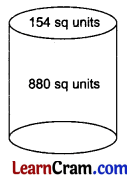Solution:
The surface area of the circular face of the cylinder = 154 sq. units (Given)
⇒ πr2 = 154
⇒ $$\frac{22}{7}$$ × r2 = 154
⇒ r2 = $$\frac{154 \times 7}{22}$$ = 49
⇒ r = 7 units
The lateral surface area of the cylinder = 880 sq. units
⇒ 2πrh = 880
⇒ 2 × $$\frac{22}{7}$$ × 7 × h = 880
⇒ h = $$\frac{880}{2 \times 22}$$
⇒ h = 20 units
Thus, the radius of the cylinder = 7 units, and the height = 20 units.Question 2.
The length of a room is 50 percent more than its breadth. The cost of carpeting the room at the rate of ₹ 38.50 per m2 is ₹ 924 and the cost of painting the walls at the rate of ₹ 5.50 per m2 is ₹ 1,320. Find the dimensions of the room.
Solution:
Let the breadth of the room, i.e., b be x m.
Then, the length of the room, i.e., l = x + x × $$\frac{50}{100}$$ = $$\frac{3x}{2}$$ m
Area of the floor of the room = $$\frac{924}{38.50}$$
⇒ l × b = 24 m2
⇒ $$\frac{3x}{2}$$ × x = 24
⇒ 3x2 = 48
⇒ x = 4 m
∴ Length of the room = 6 m
and breadth of the room = 4 m
Area of the room to be painted = $$\frac{1320}{5.50}$$ = 240 m2
Area of four walls = 240
⇒ 2(l + b) × h = 240
⇒ 2(6 + 4) × h = 240
⇒ 2 × 10 × h = 240
⇒ h = 12 m
Thus, length = 6 m, breadth = 4 m and height = 12 m

### DAV Class 8 Maths Chapter 1 Enrichment Questions

Question 1.
This is a net of a triangular prism.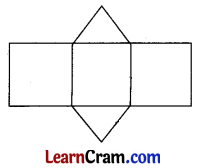Can you tell me the 3D shape that you will get using the given net?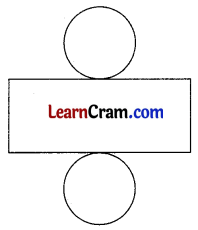Draw the net of-
(i) a triangular pyramid.
(ii) a square prism.
(iii) a cone.
Solution:We will get the right circular cylinder using the net shown alongside.
(i) Net of a triangular pyramid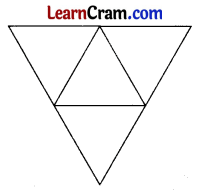(ii) Net of a square prism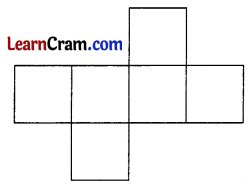(iii) Net of a cone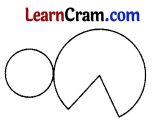Question 1.
Find the area of a quadrilateral one of whose diagonal is 25 cm long and the lengths of perpendiculars from the other two vertices are 16.4 cm and 11.6 cm respectively.
Solution: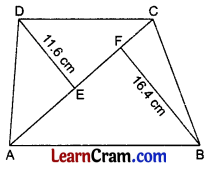Area of ABCD = $$\frac{1}{2}$$ × AC × (DE + BF)
= $$\frac{1}{2}$$ × 25 × (11.6 + 16.4)
= $$\frac{1}{2}$$ × 25 × 28
= 350 cm2
Hence, the required area = 350 cm2Question 2.
Calculate the area of quadrilateral ABCD in which ABCD is an equilateral triangle with each side equal to 26 cm, ∠BAD = 90°, and AD = 24 cm.
Solution:In ΔABD,
AB2 + AD2 = DB2 [By Pythagoras Theorem]
⇒ AB2 + (24)2 = (26)2
⇒ AB2 + 576 = 676
⇒ AB2 = 676 – 576
⇒ AB2 = 100
⇒ AB = 10
Area of DABCD = area of ΔDAB + area of ΔDCB
= $$\frac{1}{2}$$ × AB × AD + $$\frac{\sqrt{3}}{4}$$ × DB2
= $$\frac{1}{2}$$ × 10 × 24 + $$\frac{\sqrt{3}}{4}$$ × (26)2
= 120 + 169√3
= 120 + 169 × 1.732
= 120 + 292.37
= 412.37 cm2

Question 3.
The two parallel sides of a trapezium are 58 m and 42 m long. The other two sides being equal are 17 m, find its area.
Solution: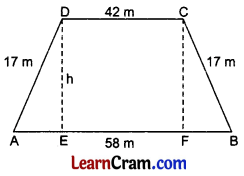Draw DE ⊥ AB and CF ⊥ AB.
∴ DC = EF = 42 m
and AE = FB = $$\frac{58-42}{2}$$ = 8 m
In right ΔAED,
AE2 + ED2 = AD2 [By Pythagoras Theorem]
⇒ (8)2 + h2 = (17)2
⇒ 64 + h2 = 289
⇒ h2 = 289 – 64
⇒ h2 = 225
⇒ h = 15 m
∴ Area of the trapezium ABCD = $$\frac{1}{2}$$ × (AB + DC) × h
= $$\frac{1}{2}$$ × (42 + 58) × 15
= $$\frac{1}{2}$$ × 100 × 15
= 750 m2
Hence, the required area = 750 m2Question 4.
The adjoining figure shows a field with the measurement given in meters. Find the area of the field.
Solution: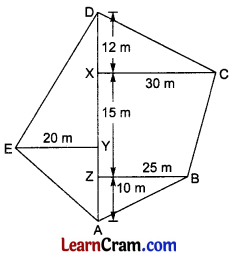Area of the given field = ar(ΔAZB) + ar(Trap. ZBCX) + ar(ΔDXC) + ar(ΔDAE)
= [$$\frac{1}{2}$$ × 10 × 25] + [$$\frac{1}{2}$$ × (30 + 25) × 15] + [$$\frac{1}{2}$$ × (30 × 12] + [$$\frac{1}{2}$$ × (12 + 15 + 10) × 20]
= 125 + 412.5 + 180 + 370
= 1087.5 m2
Hence, the required area = 1087.5 m2

Question 5.
Find the volume, surface area, and lateral surface area of a cuboid whose length is 8.5 m, breadth is 6.4 m, and height is 50 cm.
Solution:
Here, l = 8.5 m, b = 6.4 m, h = 50 cm = $$\frac{1}{2}$$ m
Volume = l × b × h
= 8.5 × 6.4 × $$\frac{1}{2}$$
= 27.2 m3
Surface area = 2[lb + bh + lh]
= 2[8.5 × 6.4 + 6.4 × $$\frac{1}{2}$$ + $$\frac{1}{2}$$ × 8.5]
= 2[54.4 + 3.2 + 4.25]
= 2 × 61.5
= 123.70 m2
Lateral surface area = 2[l + b] × h
= 2[8.5 + 6.4] × $$\frac{1}{2}$$
= 14.9 m2
Hence, the required volume = 27.2 m3
Surface area = 123.70 m2
Lateral surface area = 14.9 m2

Question 6.
Find the length of the longest rod that can be placed in a room measuring 12 m × 9 m × 8 m.
Solution:
Length of the longest rod = Length of the diagonal
= $$\sqrt{l^2+b^2+h^2}$$
= $$\sqrt{(12)^2+(9)^2+(8)^2}$$
= $$\sqrt{144+81+64}$$
= $$\sqrt{289}$$
= 17 m
Hence, the required length = 17 mQuestion 7.
The volume of a cuboid is 14400 cm3 and its height is 15 cm. The cross-section of the cuboid is a rectangle having its sides in the ratio 5 : 3. Find the perimeter of the base.
Solution:
Let the length be 5x m and breadth be 3x m.
∴ Volume of cuboid = (Area of cross-section) × height
⇒ 14400 = (5x × 3x) × 15
⇒ 14400 = 225x2
⇒ x2 = 64
⇒ x = 8 cm
Length = 5 × 8 = 40 cm
Breadth = 3 × 8 = 24 cm
∴ Perimeter of base = 2[l + b]
= 2[40 + 24]
= 2 × 64
= 128 cm
Hence, the required perimeter = 128 cm.

Question 8.
The sum of the length, breadth, and depth of a cuboid is 19 cm and the length of its diagonal is 11 cm. Find the surface area of the cuboid.
Solution:
l + b + h = 19 and $$\sqrt{l^2+b^2+h^2}$$ = 11
⇒ l2 + b2 + h2 = 121
(l + b + h)2 = (19)2
⇒ l2 + b2 + h2 + 2lb +2bh + 2lh = 361
⇒ 121 + 2[lb + bh + 2lh] = 361
⇒ 2[lb + bh + lh] = 361 – 121 = 240 m2
Hence, the total surface area = 240 m2

Question 9.
Three cubes each of side 6 cm, are joined end to end. Find the surface area of the resulting cuboid.
Solution:
Length of the resulting cuboid = 6 × 3 = 18 cm
breadth = 6 cm and height = 6 cm
∴ Surface area = 2[lb + bh + lh]
= 2[18 × 6 + 6 × 6 + 6 × 18]
= 2[108 + 36 + 108]
= 2 × 252
= 504 cm2
Hence, the required surface area = 504 cm2Question 10.
A cylindrical tank has a capacity of 6160 m3. Find its depth if its radius is 14 m. Also, find the cost of painting on a curved surface at ₹ 3 per m2.
Solution:
Volume = πr2h
⇒ 6160 = $$\frac{22}{7}$$ × 14 × 14 × h
⇒ h = $$\frac{6160 \times 7}{22 \times 14 \times 14}$$
⇒ h = 10 m
Curved surface area = 2πrh
= 2 × $$\frac{22}{7}$$ × 14 × 10
= 880 m2
Cost of painting of its curved surface = 880 × ₹ 3 = ₹ 2640
Hence, the required depth is 10 m, and the required cost = ₹ 2640

Question 11.
The sum of the radius of the base and the height of a cylinder is 37 m. If the total surface area of the cylinder is 1628 m2, find its volume.
Solution:
Given that (r + h) = 37 m
and total surface area = 1628 m2
∴ T.S.A. = 2πr(h + r)
⇒ 1628 = 2 × $$\frac{22}{7}$$ × r × 37
⇒ r = $$\frac{1628 \times 7}{2 \times 22 \times 37}$$
⇒ r = 7 m
∵ r + h = 37
⇒ 7 + h = 37
⇒ h = 37 – 7 = 30 cm
∴ Volume = πr2h
= $$\frac{22}{7}$$ × 7 × 7 × 30
= 4620 cm3
Hence, the required volume = 4620 m3Question 12.
A swimming pool 70 m long, 44 m wide, and 3 m deep is filled by water issuing from a pipe of diameter 35 cm, at 6 m per second. How many hours does it take to fill the pool?
Solution:
The volume of the swimming pool = l × b × h
= 70 × 44 × 3
= 210 × 44
= 9240 m3
The volume of water issuing from the pipe in 1 sec = πr2h
= $$\frac{22}{7} \times \frac{35}{2 \times 100} \times \frac{35}{2 \times 100}$$ × 6 m3
∴ Volume of water flowing into swimming pool = $$\frac{22}{7} \times \frac{35}{2 \times 100} \times \frac{35}{2 \times 100}$$ × 6 × 60 × 60 m3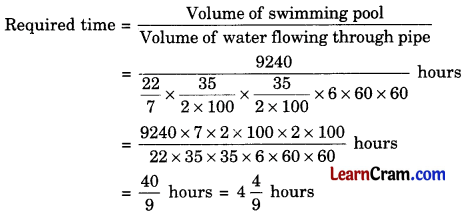Hence, the required time = $$4 \frac{4}{9}$$ hours.